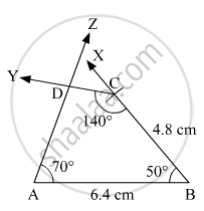Advertisement Remove all ads

# Construct the Following Quadrilateral of Given Measures. in ☐ Abcd L(Ab) = 6.4 Cm, L(Bc) = 4.8 Cm, M∠A = 70° , M∠B = 50° , M∠C = 140°. - Mathematics

Sum

Construct the following quadrilateral of given measures.

In ☐ ABCD l(AB) = 6.4 cm, l(BC) = 4.8 cm, m∠A = 70° , m∠B = 50° , m∠C = 140°.

Advertisement Remove all ads

#### Solution

Steps of Construction:

Step 1:
Draw AB = 6.4 cm.

Step 2: Draw ∠ABX = 50° .

Step 3: With B as centre and radius 4.8 cm, draw an arc cutting ray BX at C.

Step 4: Draw ∠BCY = 140° .

Step 5: Draw ∠BAZ = 70° .

such that ray AZ and ray CY intersect each other at D.Here, ABCD is the required quadrilateral.

Is there an error in this question or solution?
Advertisement Remove all ads

#### APPEARS IN

Balbharati Mathematics 8th Standard Maharashtra State Board
Chapter 8 Quadrilateral : Constructions and Types
Practice Set 8.1 | Q 3 | Page 43
Advertisement Remove all ads
Advertisement Remove all ads
Share
Notifications

View all notifications

Forgot password?